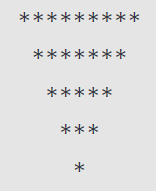Related Tags

javascript
communitycreator

# How to generate a reverse pyramid pattern in JavaScript

Ayush Trivedi

### Overview

In this shot, we will learn how to print a reverse pyramid pattern in JavaScript.

We will use 3 for loops; one is the outer loop for the rows of the pyramid and the other two are nested loops. One inner/nested loop will print the number of spaces, and the other will print the star (*).

Below is the pattern we are going to print.### Code

Let’s see the program to create a pyramid pattern.

let n = 5;
// External loop
for (let i = 0; i < n; i++) {
// printing spaces
for (let j = 0; j < i; j++) {
process.stdout.write(' ')
}
// printing star
for (let k = 0; k < 2 * (n-i) - 1; k++) {
process.stdout.write('*')
}
console.log();
}
Code to print pyramid pattern

### Code explanation

• In line 1, we initialize a number that is the height of the triangle (number of rows).

• In line 3, we have our outer for loop, which will iterate over the triangle’s height, which is 5.

• In line 5, we have the first inner for loop to print spaces for i times, where i is the outer loop iteration number. For example, if i=3 (for the 4th row), the number of spaces before the star will be 2, for i=4, the number of spaces will be 3, and so on.

• In line 6, we use the standard method of JavaScript, process.stdout.write(' '), to print the space.

• In line 9, we have the second inner for loop to print * for 2 * (n - i) - 1 times (where i is the current iteration number of the outer loop). For example, if i=3 (for the 4th row), after 3 spaces, it will print 3 stars (2 * (5 - 3) - 1).

• In line 10, we use process.stdout.write() to print the star.

• In line 12, we use console.log() with null, as it will change to a new line. We can use process.stdout.write(’\n’) to change the line.

### Output

We have completed the task to print * in the form that looks like a reverse (downward) pyramid.

RELATED TAGS

javascript
communitycreator

CONTRIBUTOR

Ayush Trivedi
RELATED COURSES

View all Courses

Keep Exploring

Learn in-demand tech skills in half the time